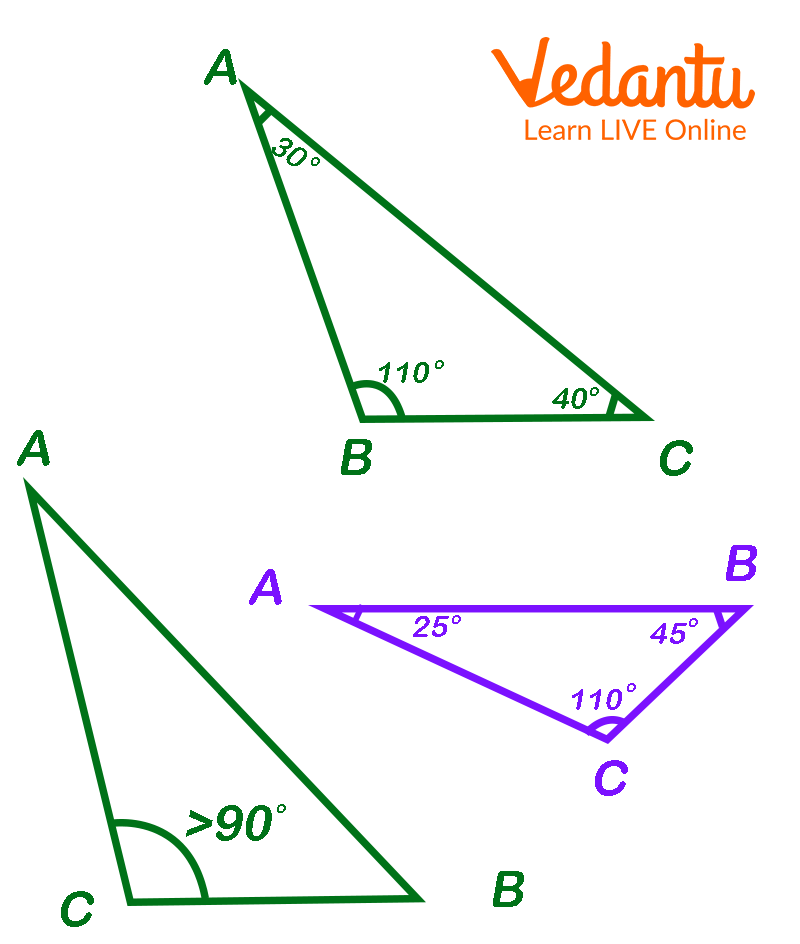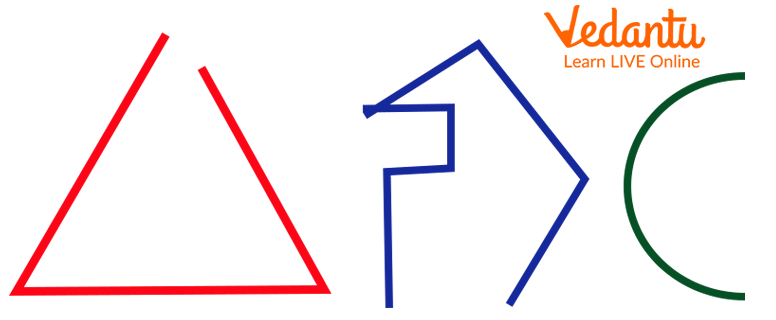Courses
Courses for Kids
Free study material
Free LIVE classes
MoreLIVE
Join Vedantu’s FREE Mastercalss

1, 2, 3, 4, 5, …… You can read these numbers easily, right? What is the first number? It is ONE— o-n-e, one. Isn’t it? Maths starts with the number “1”. Now, you can imagine how important the letter ‘O’ is in Mathematics.

Though the numbers start with 0, can you recall the number series taught to you in your school? Or, how did your mother teach you counting? So, in general, the counting begins with “1”.

Many other important words begin with the alphabet O. A list of Maths words that start with O is given below. Add them to your vocabulary and become a pro in Mathematics.

## Lists of Maths Words that Begin with the Letter ‘O’

1. One: One is the smallest natural number. It is written as ‘1’ in numbers. It represents a single unit or quantity. It lies between 0 and 2.

2. Odd Numbers: The numbers which are not divisible by 2 are called odd numbers. The series of 1, 3, 5, 7, …. is a series of odd numbers. When these numbers are divided by 2, they leave 1 as a remainder.

3. Octagon: A plane figure which has 8 sides is called an Octagon. It has eight vertices and 8 angles. Also, the prism which is bounded by the 2 octagonal bases and 8 square sides is called an octagonal prism. It has 16 vertices and 12 edges.

4. Obtuse Angle: The angle which is greater than 90° and less than 180° is called the obtuse angle. The triangle in which one angle measures more than 90°, i.e., an obtuse angle is called an obtuse triangle.Examples of Obtuse Triangle

5. Open Figure: Unlike a closed figure like a triangle, or quadrilateral, there are some figures in which one of the line segments or curves do not meet. These figures do not have any starting or ending point. Some examples of the open figure are shown in the below figure.Example of Open Figure

6. Ounce: In general, an ounce is referred to as a very small amount of something. In the United States, it is used to measure weight. There are 16 ounces in the pound and it is equivalent to 28.35 grams. Ounces is abbreviated as oz.

7. Origin: Origin is the point from which coordinates are measured. It is the point at which the X- and Y-axis meet and is generally considered as (0, 0).

8. Order: Order shows the arrangement of different items or sequences with each other following a particular pattern or sequence. Also, the degree of an equation, expression, etc. is denoted by an ordinal number. In matrices, the order is referred to as the number of rows and columns. In the differential equation, the order of an equation is referred to as the number of differentiation required to reach the highest derivatives.

## Do You Know?

• The sum of two odd numbers is always an even number whereas the product of two odd numbers always results in an odd number.

• Number 0 is an even number.

## Conclusion

Last updated date: 30th Sep 2023
Total views: 132.9k
Views today: 1.32k

1. What are odd numbers in Mathematics?

The numbers which are not divisible by 2 are called odd numbers. We can also say that any number in which the one digit is 1, 3, 5, 7, or 9 is called an odd number. The series of odd numbers are the following:

1, 3, 5, 7, 9, 11…..

Except 1, when these numbers are divided by 2, it leaves 1 as a remainder. Odd numbers can never be divided into exactly two pairs.

2. What is an obtuse triangle?

Like any other triangle, the obtuse triangle has three sides, three vertices, and three angles. But in the obtuse triangle, one of the angles is greater than 90°.

In such a triangle, no angle could be equal to 0° or 180°  and it could not be more than 180° (as the sum of all angles of a triangle is equal to 180°).